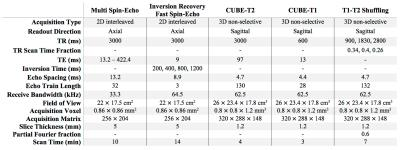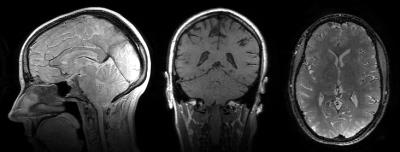T1-T2 Shuffling: Multi-Contrast 3D Fast Spin-Echo with T1 and T2 Sensitivity
Jonathan I Tamir1, Valentina Taviani2, Shreyas S Vasanawala3, and Michael Lustig1

1Electrical Engineering and Computer Sciences, University of California, Berkeley, Berkeley, CA, United States, 2MR Applications and Workflow, GE Healthcare, Menlo Park, CA, United States, 3Radiology, Stanford University, CA, United States

### Synopsis

Volumetric fast spin-echo (3DFSE) imaging is clinically desirable because of its robustness to off-resonance and its utility for obtaining many types of image contrasts at isotropic resolution. However, its routine clinical use is inhibited by blurring due to long echo trains needed to maintain scan efficiency. Her we present T1-T2 Shuffling, a 3DFSE-based acquisition and reconstruction scheme that mitigates image blur and retrospectively synthesize T1-weighted and T2-weighted image contrasts. The acquisition, an extension of T2 Shuffling, employs a randomizing echo train view ordering with variable repetition times (TRs). The use of short TRs increases scan efficiency while providing T1 sensitivity.

### Introduction

Volumetric fast spin-echo (3DFSE) imaging is clinically desirable because of its robustness to off-resonance and its utility for obtaining many types of image contrasts at isotropic resolution. However, due to image blurring resulting from long echo trains$^{1,2}$, current standard clinical practice is to separately acquire 2DFSE images (for each desired contrast and orientation) while sacrificing through-plane resolution. Recently, we introduced T2 Shuffling$^{3}$, a 3DFSE-based acquisition and reconstruction method that mitigates signal blur and resolves images at multiple virtual echo times (TEs) along the T2 relaxation curve. T2 Shuffling uses a randomized view ordering and re-acquires phase encodes throughout the echo train. The 4D data are processed with a compressed sensing-based reconstruction$^{4}$ that constrains the temporal signal evolutions to a low-dimensional subspace. In this work we extend the sequence to include T1 sensitivity by supporting a variable repetition time (TR) during the acquisition. The reconstructed subspace images representing the variable TEs and TRs are then linearly combined to synthesize arbitrary T1-weighted and T2-weighted image contrasts$^{5}$. In contrast to MR Fingerprinting$^{6}$, the signal evolution for each TR is independent and the reconstruction is non-parametric.

### Pulse Sequence Design

The CUBE 3DFSE pulse sequence (GE Healthcare) was previously modified to re-sample phase encodes at different echo times$^{3}$. Here we add the capability to specify the TR independently for each echo train to provide T1 sensitivity. Variable-density Poisson-disc sampling patterns are prescribed for each unique TE and TR combination. The signal evolution with driven-equilibrium at the $i^\mathrm{th}$ TE and the $j^\mathrm{th}$ TR is depicted in Figure 1 for different combinations of (T1,T2), and is given by$^{7}$$$S\left(\mathrm{TE}_i,\mathrm{TR}_j\right)=M_0f\left(T_1,T_2,\mathrm{TE}_i\right)\frac{1-E_1(\mathrm{TR}_j)}{1-E_1(\mathrm{TR}_j)f\left(T_1,T_2,\mathrm{TE}_T\right)},$$where $f\left(T_1,T_2,\mathrm{TE}_i\right)$ is the relaxation signal at the $i^\mathrm{th}$ TE, $E_1(\mathrm{TR})=\exp\left(-\frac{\mathrm{TR}-T{\times}T_s}{T_1}\right)$, $T_s$ is the echo spacing, and $T$ is the echo train length. The relaxation signal $f\left(T_1,T_2,\mathrm{TE}\right)$ depends on the variable refocusing flip angle scheme and can be calculated with the extended phase graph algorithm$^{2}$.

### Reconstruction Algorithm

The signal evolutions for each TR are concatenated into a single temporal curve. The forward model is $\mathbf{y}=\mathbf{Ex}$, where $\mathbf{x}$ is the time series of images at each TE and TR, $\mathbf{E}$ is the ESPIRiT$^{8}$ sensitivity encoding operator, and $\mathbf{y}$ are the k-space measurements. The model is relaxed, and the signal is approximated by $\mathbf{x}=\mathbf{\Phi\alpha}$, where $\mathbf{\Phi}$ is a pre-determined temporal basis$^{3,9}$ of dimension K and $\alpha$ are the temporal image coefficients. The reconstruction is posed as $$\min_\alpha\frac{1}{2}||\mathbf{y}-\mathbf{E\Phi\alpha}||_2^2+\lambda\sum_\mathbf{r}||R_\mathbf{r}(\alpha)||_*$$ Locally low rank regularization$^{10}$ is applied to the coefficients to enforce spatio-temporal sparsity. The temporal basis is constructed by simulating an ensemble of N signal evolutions with (T1,T2) values that match the distribution of the anatomy of interest$^{3}$. After reconstruction, the temporal coefficients are linearly combined to match a target image contrast. The linear combination is found by fitting the simulated signal evolution coefficients to a simulated image contrast:$^{5,11}$$$\min_\mathbf{c}\sum_{n=1}^N\left(g_n\left(T_1,T_2\right)-\mathbf{c}^T\alpha_n\right)^2,$$where $g_n\left(T_1,T_2\right)$ is the simulated contrast of the $n^\mathrm{th}$ signal in the ensemble and $\alpha_n$ are its temporal coefficients. For example, a spin-echo contrast is simulated through $g_n\left(T_1,T_2\right)=\exp\left(-\frac{\mathrm{TE}}{T_2}\right)\left(1-\exp\left(-\frac{\mathrm{TR}-\mathrm{TE}}{T_1}\right)\right)$. The reconstruction was implemented with the Berkeley Advanced Reconstruction Toolbox$^{12}$.

### Experiments

All experiments were performed under IRB approval with informed consent, and scan parameters are shown in Figure 2. A volunteer’s brain was scanned at 3T using conventional 2D multi spin-echo and inversion recovery FSE, and the data were used to obtain reference T1 and T2 maps$^{13}$ (Figure 3a). An ensemble of N=10240 signal evolutions was chosen from the T1-T2 distribution and a basis of size K=4 was constructed using SVD$^{3,9,14}$ (Figure 3b). The linear combinations for each desired image contrast were computed from the ensemble. The process is depicted in Figure 3c for forming proton-density, T2-weighted, and T1-weighted images. Another volunteer’s brain was scanned at 3T using CUBE-T2 and CUBE-T1 to obtain reference T2-weighted and T1-weighted images, respectively, followed by T1-T2 Shuffling with variable TR. Three TR values and their respective fractions of scan time were chosen based on the Cramer-Rao lower bound$^{13}$ of Eq. 1 for a fixed scan time of 7 minutes. The data were reconstructed according to Eq. 2 and linearly combined according to Eq. 3.

### Results and Discussion

Figure 4 shows an animated image of the reconstructed images for spin-echo, saturation recovery, and inversion recovery. The reconstruction enables full retrospective reformats of orientation and image contrast. The use of short TRs for T1 sensitivity provides high scan efficiency and makes the inverse problem more well-posed. Figure 5 shows the conventional T2 and T1 weighted images obtained from two separate CUBE acquisitions alongside the synthesized images from T1-T2 Shuffling. There is good agreement between the conventional and synthesized images.

### Conclusion

T1-T2 Shuffling provides 3D-reformattable, multi-contrast images in clinically feasible scan times.

### Acknowledgements

We thank the following funding sources: NIH Grants R01EB019241, R01EB009690, and P41RR09784, Sloan Research Fellowship, Okawa Research Grant, and GE Healthcare.

### References

1. Mugler JP. Optimized Three-dimensional Fast-spin-echo MRI. Journal of magnetic resonance imaging 39, no. 4 (2014). doi:10.1002/jmri.24542.

2. Busse RF, Hariharan H, Vu A, Brittain JH. Fast Spin Echo Sequences with Very Long Echo Trains: Design of Variable Refocusing Flip Angle Schedules and Generation of Clinical T2 Contrast. Magnetic resonance in medicine 55, no. 5 (2006). doi:10.1002/mrm.20863.

3. Tamir JI, Uecker M, Chen W, Lai P, Alley MT, Vasanawala SS, Lustig M. T2 Shuffling: Sharp, Multicontrast, Volumetric Fast Spin-echo Imaging. Magnetic resonance in medicine (2016). doi:10.1002/mrm.26102. [Epub ahead of print].

4. Lustig, M, Donoho D, Pauly JM. Sparse MRI: The Application of Compressed Sensing for Rapid MR Imaging. Magnetic resonance in medicine 58, no. 6 (2007). doi:10.1002/mrm.21391.

5. Warntjes, JBM, Leinhard OD, West J, Lundberg P. Rapid Magnetic Resonance Quantification on the Brain: Optimization for Clinical Usage. Magnetic resonance in medicine 60, no. 2 (2008). doi:10.1002/mrm.21635.

6. Ma D, Gulani V, Seiberlich N, Liu K, Sunshine JL, Duerk JL, Griswold MA. Magnetic Resonance Fingerprinting. Nature 495, no. 7440 (2013). doi:10.1038/nature11971.

7. Malik, SJ, Beqiri A, Padormo F, Hajnal JV. Direct Signal Control of the Steady-state Response of 3D-FSE Sequences. Magnetic resonance in medicine 73, no. 3 (2015). doi:10.1002/mrm.25192.

8. Uecker M, Lai P, Murphy MJ, Virtue P, Elad M, Pauly JM, Vasanawala SS, Lustig M. ESPIRiT-an Eigenvalue Approach to Autocalibrating Parallel MRI: Where SENSE Meets GRAPPA. Magnetic resonance in medicine 71, no. 3 (2014). doi:10.1002/mrm.24751.

9. Huang C, Graff CG, Clarkson EW, Bilgin A, Altbach MI. T2 Mapping From Highly Undersampled Data by Reconstruction of Principal Component Coefficient Maps Using Compressed Sensing. Magnetic resonance in medicine 67, no. 5 (2012). doi:10.1002/mrm.23128.

10. Trzasko JD, Manduca A. Local versus global low-rank promotion in dynamic MRI series reconstruction. In Proceedings of International Symposium on Magnetic Resonance in Medicine, Vol. 19, Montreal, Quebec, Canada, 2011. p. 4371.

11. Vidarsson L, Conolly SM, Lim KO, Gold GE, Pauly JM. Echo Time Optimization for Linear Combination Myelin Imaging. Magnetic resonance in medicine 53, no. 2 (2005). doi:10.1002/mrm.20360.

12. Uecker M, Tamir JI, Ong F, Iyer S, Cheng JY, Lustig M, BART: version 0.3.01. Zenodo (2016). doi: 10.5281/zenodo.50726

13. Barral, JK, Gudmundson E, Stikov N, Etezadi-Amoli M, Stoica P, Nishimura DG. A Robust Methodology for in Vivo T1 Mapping. Magnetic resonance in medicine 64, no. 4 (2010). doi:10.1002/mrm.22497.

14. Zhao B, Setsompop K, Gagoski B, Ye H, Adalsteinsson E, Grant PE, Wald LL. A model-based approach to accelerated magnetic resonance fingerprinting time series reconstruction. In Proceedings of International Symposium on Magnetic Resonance in Medicine, Vol. 24, Singapore, 2016. p. 0871.

### Figures(a) Simulated steady-state transverse and longitudinal magnetization signal evolution curves for FSE with driven equilibrium across a TR of 1500 ms using variable-refocusing flip angles shown in (b). Each curve represents a different (T1, T2) pair.Scan and imaging parameters used for the in vivo experiments. All scans were performed at 3T with IRB approval and informed consent.(a) Fully sampled scans of 2D multi spin-echo and inversion recovery fast spin-echo were used to estimate T1 and T2 maps of the brain at 3T. These maps were used to derive a T1-T2 distribution and simulate an ensemble of signal evolutions with variable refocusing flip angles and variable TRs. (b) The signal evolutions at each TR were concatenated and a subspace was formed through SVD. (c) Using the subspace and the known T1 and T2 values, a linear combination was found to synthesize arbitrary image contrasts.Reconstructed spin-echo image animations at different contrasts and orientations. Left: sagittal reformat at a TR of 7000 ms and TE increasing from 5 ms to 200 ms shows the T2 relaxation curve. Middle: coronal reformat at TE = 10 ms and TR increasing from 200 ms to 7000 ms shows the T1 saturation recovery curve. Right: Axial reformat at TE = 10 ms, TR = 7000 ms, and TI increasing from 50 ms to 7000 ms shows the T1 inversion recovery curve.Comparison of conventional T1-weighted and T2-weighted images obtained from two separate CUBE acquisitions with synthesized image contrasts using T1-T2 Shuffling (single acquisition).